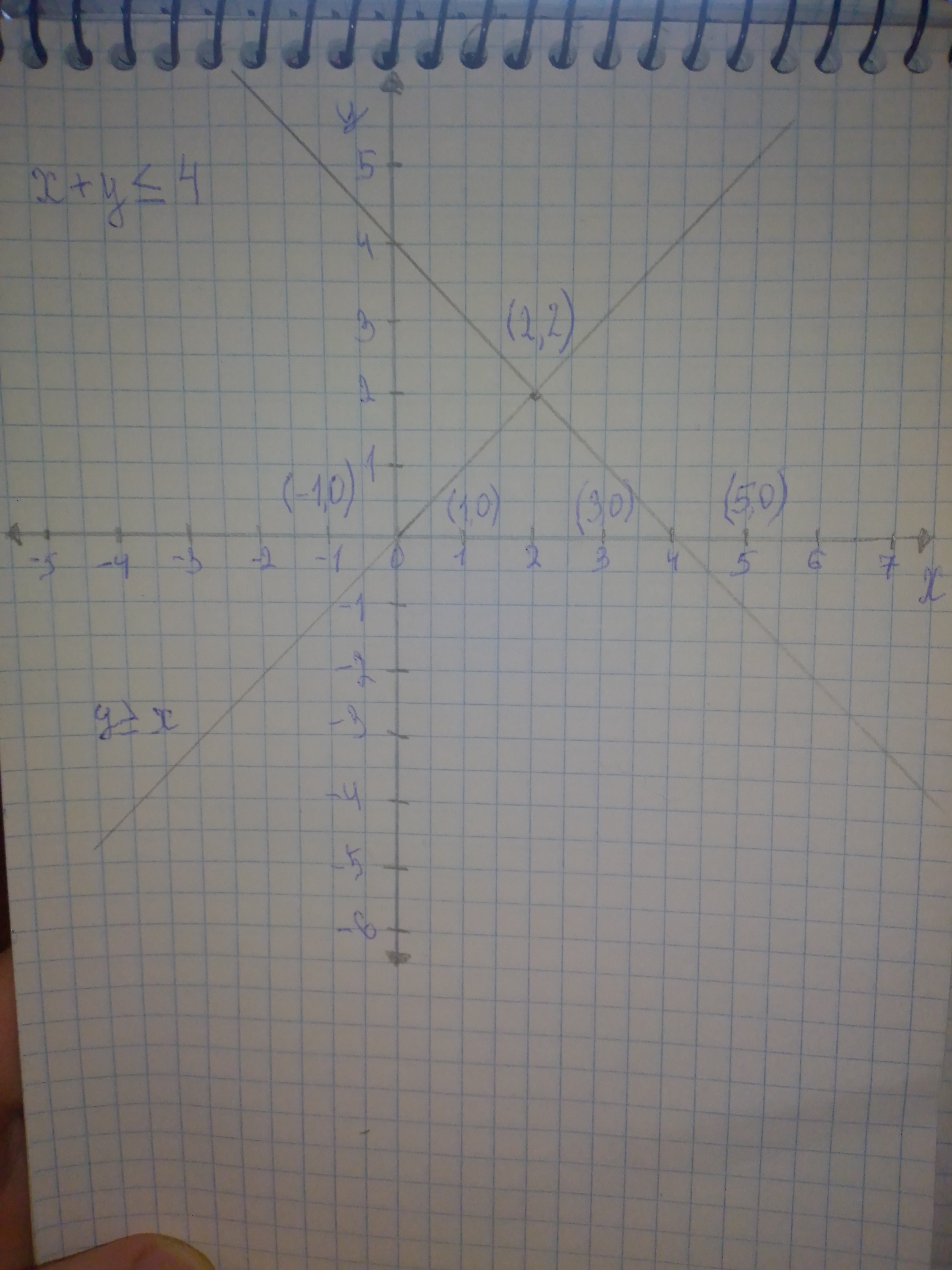# Systems of inequalities graph the solutions set of the systemusagirl007A 2020-12-28 Answered

Systems of inequalities graph the solutions set of the system if inequalities. Find the coordinates of all vertices, and determine whether the solution set is bounded. $\left\{\begin{array}{l}x+y\le 4\\ y\ge x\end{array}$

You can still ask an expert for help

• Questions are typically answered in as fast as 30 minutes

Solve your problem for the price of one coffee

• Math expert for every subject
• Pay only if we can solve itwornoutwomanC

Graph: The given system of the inequalities is, $x+y\le 4\dots ..\left(1\right)$
$y\ge x\dots ..\left(2\right)$ The corresponding equation of the inequality (1) is, $x+y=4$
$y=4-x$ Since, the inequality $x+y\le 4$ involvesthe less than or equal to sign, then the points on the line $y=4-x$ satisfies the inequality $x+y\le 4$. Therefore, the line $y=4-x$ itself is the part of the solution set. Consider the test points (3,0) and (5,0) that lies on either side of the line $y=4-x$, to check whether the solutions of the inequality $x+y\ge 2$ is on right side or left side. Substitute 3 for x and 0 for y in and the inequality $x+y\le 4$.

$3\le 4$ The point (3,0) is on the left side of the line and satisfies the inequality $x+y\le 4$. So it is not the part of the solution set. Substitute 5 for x and 0 for y in and the inequality $x+y\le 4$.

$5n\le 4$ The point (5,0) is on the right side of the line and does not satisfy the inequality $x+y\le 4$. So it is the part of solution set. The corresponding equation of the inequality (2) is, $x=y$ Since, the inequality $y\ge x$ involves the greater than or equal to sign, then the points on the line $x=y$ satisfies the inequality $y\ge x$ Therefore, the line $x=y$ itself is the part of the solution set. Consider the test points (0,1) and (1,0) that lies on either side of the line $x=y$, to check whether the solutions of the inequality $y\ge x$ is on right side or left side. Substitute 0 for x and 1 for y in the inequality $y\ge x$. ${y}_{\ge }^{?}x$
$1\ge 0$ The point (0,1) is on the left side of the line and satisfies the inequality $x-y\ge 0$. So it is the part of the solution set. Substitute 1 for x and 0 for y in the inequality $x-y\ge 0$. ${y}_{\ge }^{?}x$
$0n\ge$ The point (1,0) is on the right side of the line and does not satisfy the inequality $x-y\ge 0$. So it is the part of solution set. The solution set of the given system of inequalities is the intersection of the solutions of each of the given inequality. Therefore, the solution set is shown as shaded region.Calculation: The vertices occur at the points of intersection of the corresponding equation of the given system of inequalities. $x+y=4\dots ..\left(3\right)$
$x=y\dots ..\left(4\right)$ Substitute y for x in equation (3). $x+y=4$
$x+x=4$
$2x=4$
$x=2$ Therefore, the x-coordinate of vertex is 2. Now subtitute 2 for x in equation (4) to evaluate the y-coordinate of vertex. $y=x$
$y=2$ The vertex of given system of inequalities is (2,2). It is observed that the shaded region is not enclosed by the boundary lines of the given system of inequalities. Therefore, the shaded region is not bounded or unbounded. Interpretation: The solution set of the given system of inequality lies in the I quadrant and IV quadrant. Answer: Thus, the vertex of the given system of inequalities is (2,2) and the solution set is unbounded.